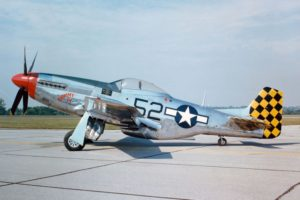1

# Quadrotor Dynamic Model: Propeller Gyroscopic Effect.

The equations below are from the last post, where we see we matched our Bouabdallah paper‘s equations for rotational motion.

We know some propeller input is going to be a part of the applied torque. There is going to be a set of equations for linear motion too, but let’s clarify what is going on here regarding torques about our 3 body axes. Let’s look at these equations and…

# Review Rigid body rotation gyroscopic effectRemember Newton’s Second Law? This tells us that the sum of the forces on a mass in the i’th direction must equal the mass of the body times the acceleration in that direction.

If we take one of the equations above and rewrite it we can recognize this familiar form, albeit in rotational nomenclature. The rightmost term is the gyroscopic effect of the entire rigid body. It tells us that if there is rotational rate about y- and z-axes a torque is induced about the X-axis. If we neglect this term you see we’d be left within simple second-law form.

It’s a little confusing, but all we’ve done is already offer one item to the, “sum-of-torques”: the gyroscopic effect resulting from the rigid body rotation. Notice when we moved the term in parenthesis to the other side it looks like an added torque now but we swapped the subtraction in parenthesis so the math is the same.

# Gyroscopic effect of Propellers rotation.

## “tail-dragger” airplane example

The video below to describes a phenomenon familiar to pilots, particularly pilots of powerful, “tail draggers” like the P-51 Mustang. A propeller is a large spinning disk. When we tip the plane of rotation of the propeller: pitch the airplane, a torque on the body results due to this gyroscopic effect.Imagine you are in the cockpit of the P-51, speeding down the runway (lucky you). That monster prop has a healthy angular momentum vector pointing perpendicular to the prop. Your tail is dragging until you get enough lift from your wings (due to streamline curvature, if you recall a recent post!). At some point your tail will lift and you’ll be parallel to the ground before your front wheels leave the ground. This looks like a quick, “pitch” of say 15-degrees based on the photo above. Let’s say it took a second for the tail to rise as we’re nearing take-off, so the pitch rate is 15-degrees per-second as the tail wheel leaves the ground.

That same pitch is pitching the plane of the spinning propeller forward, or more to the vertical. When you watch the video below and inspect the equations below you will see that this, “pitch forward” of the propeller at the above angular rate of the airplane going from dragging-to-level results in a gyroscopic torque about the airplane’s vertical axis: you feel the plane want to yaw (twist) so you’ll need to correct with some rudder.

## The effect can be negligible

Our propellers are horizontal in the body-plane, but there is a torque induced on the body that results from propeller rotation and rotation of the propeller plane about the x- and y-axis of our system too…at least in theory. It may be negligible depending on propeller moment of inertia relative to other effects, but let’s model it anyway.

We can choose to include it or neglect it later when we perform a sensitivity analysis. This is where we can look at rough magnitude of various effects and decide to include them in our model or cancel the terms in the equations: ignore them as too small to make a difference. We’ll do this systematically and not guess at this point. Besides, this is a neat phenomenon for us to appreciate in detail.

# Gyroscopic effect of our propellers

The following PDF derivations arrive at the same terms as the Bouabdallah paper (equation 8). There’s a sign convention difference between the paper and these derivations, but the result is the same:The first equation tells us that there will be a torque about our body X-axis when there is angular rate-of-change to our pitch:(not the dot, implying pitch rate). This means our quadcopter will feel a  body roll-inducing torque when it pitches. This is due to the gyroscopic effects of the 4 propellers.

The second equation tells us that there will be a torque about our body Y-axis when there is angular rate-of-change to our roll:. This means our quadcopter will feel a  body pitch-inducing torque when it rolls.  Again, this is due to the gyroscopic effects of the 4 propellers.

The hand calculations below arrive at the above equations following the process in the video. The video above explains how to go about the hand calculations for a single propeller.

## Hand Calculation PDF Notes

RB 2018-07-11 17.27.01

# Conclusion

If you expand these equations you’ll see I just performed the steps in the above video Eight times and combined the equations. We need eight steps because we have 4 propellers and we need to account for the plane-of-rotation rate-of-change about both the x- and y-axis. Rotation about the body z-axis contributes no gyroscopic effect from the propellers because it is just like twisting the axis of the propeller. The plane-of-rotation of the propeller does not change in this case so no torque is induced on the body.We’ve now accounted for gyroscopic effect of the rigid body rotations and the propeller rotations.

Next we’ll add the propeller thrust components. These will be our inputs to counteract the above effects and unknown disturbances (wind) in order to maintain the attitude of our quadcoptor and move it where we want it to go.